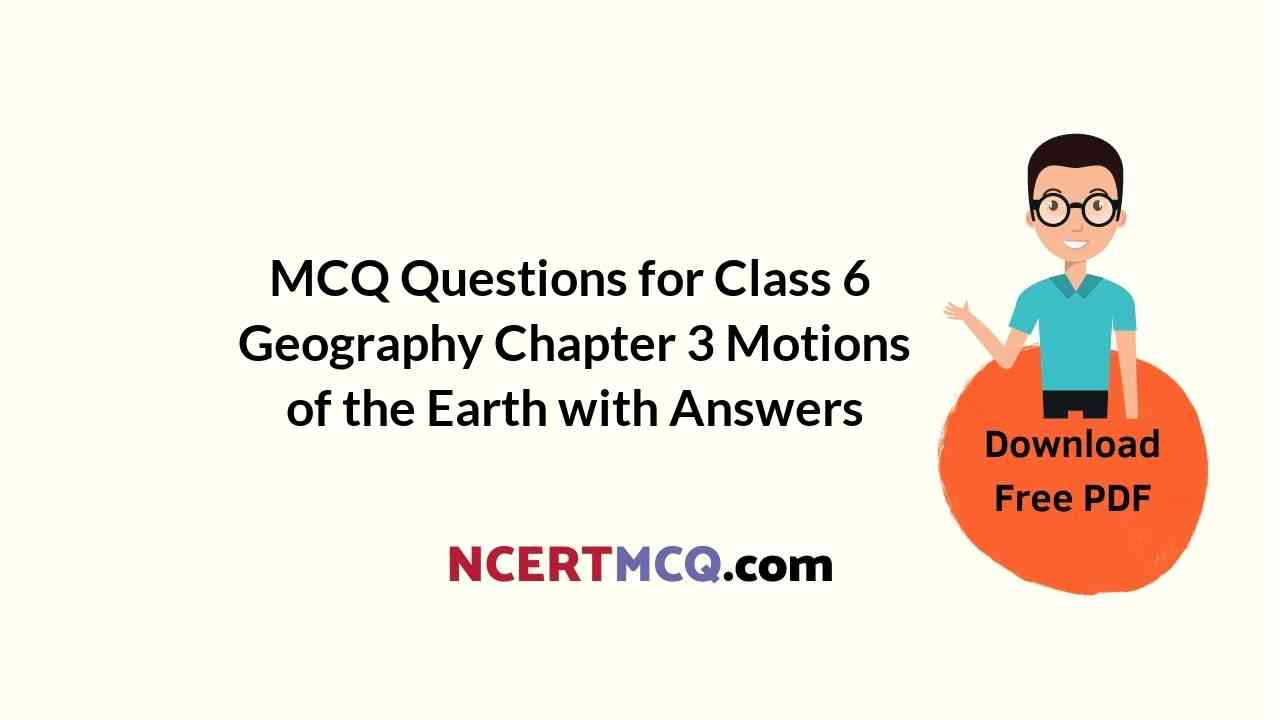Check the below NCERT MCQ Questions for Class 6 Geography Chapter 3 Motions of the Earth with Answers Pdf free download. MCQ Questions for Class 6 Social Science with Answers were prepared based on the latest exam pattern. We have Provided Motions of the Earth Class 6 Geography MCQs Questions with Answers to help students understand the concept very well.

## Motions of the Earth Class 6 MCQs Questions with Answers

Motions Of The Earth Class 6 MCQ Question 1.
Motion of the earth on its axis in about 24 hours is called
(a) revolution
(b) rotation
(c) both (a) and (b)
(d) none of these

Class 6 Geography Chapter 3 MCQ Question 2.
Motion of the earth around the sun is known as
(a) revolution
(b) rotation
(c) both (a) and (b)
(d) none of these

MCQ Questions For Class 6 Geography Chapter 3 Question 3.
What is orbital plane?
(a) Plane formed by the axis
(b) Plane formed by the orbit
(r) Both (a) and (b)
(d) None of these

Answer: (b) Plane formed by the orbit

Motion Of The Earth Class 6 MCQ Question 4.
Which one of the following is the source of light on the earth?
(a) The moon
(b) The sun
(c) The satellite
(d) The space

Ncert Class 6 Geography Chapter 3 MCQ Question 5.
The circle that divides the globe into day and night is called
(a) circle of darkness
(b) circle of day and night
(c) circle of illumination
(d)none of these

Geography Class 6 Chapter 3 MCQ Question 6.
The period of one rotation of the earth is known as
(a) the sun day
(b) the moon day
(c) the earth day
(d) none of these

Class 6th Geography Chapter 3 MCQ Question 7.
What would have happened if the earth did not rotate?
(a) Cold conditions on earth’s half portion
(b) Warm conditions on earth’s another half portion
(c) No life possible in such extreme conditions
(d) All of these

Class 6 Geography Ch 3 MCQ Question 8.
A year with 366 days is called
(a) leap year
(b) normal year
(c) both (a) and (b)
(d) none of these

MCQ Class 6 Geography Chapter 3 Question 9.
Why do seasons change on the earth?
(a) Due to change in the position of the earth around the sun
(b) Due to no change in the earth’s position
(c) Both (a) and (b)
(d) None of the above

Answer: (a) Due to change in the position of the earth around the sun

MCQ Of Motion Of The Earth Class 6 Question 10.
When do the longest day and the shortest night occur in the northern hemisphere?
(a) June 21
(b) September 23
(c) December 22
(d) March 21

Class 6 Chapter 3 Geography MCQ Question 11.
In which season Christmas is celebrated in Australia?
(a ) Winter season
(b) Summer season
(c) Autumn season
(d) Spring season

Geography Chapter 3 Class 6 MCQ Question 12.
When do equinoxes occur on the earth?
(a) March 21
(b) September 23
(c) Both (a) and (b)
(d) None of these

Answer: (c) Both (a) and (b)

Chapter 3 Geography Class 6 MCQ Question 13.
Days and nights occur on earth due to
(a) rotation
(b) revolution
(c) both (a) and (b)
(d) none of these

Motions Of The Earth Class 6 MCQ Quiz Question 14.
Change of seasons occurs on earth due to
(a) rotation
(b) revolution
(c) both (a) and (b)
(d) none of these